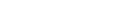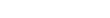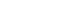# How to: handle capacity constraints for different commodities measured in different units

I have been working on a vehicle routing problem in which the truck transports several commodities, but these are measured in different units. Hence, we know the capacity of the truck for each of these commodities alone, but we don’t know how to convert them into one another so that we can load two commodities and still respect the capacity.

# How to linearize max, min, and abs functions

In this post you will see how to linearize max functions, min functions, and absolute value functions. These can be expressed mathematically as: